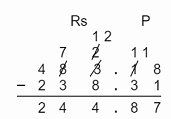### Money - Worksheets

CBSE Worksheet-01

Class - IV Mathematics – Money

Time Allowed: 30 minutes

1. Fill in the blanks with suitable words:
1. The cost of 5 pens is 60, the cost of one pen is _______.

2. _____ paise = $\frac{1}{5}$ rupees.

3. If the price of 1 television is 2032.35 rupees then the Price of 11 television is ______rupees.

4. 5 years 3 months = ________ months

5. In India the unit of currency is _____

2. State whether each of the following statement is True or False:
1. Rahul has Rs. 775.76, he gives Rs. 200 to his cousin then the amount left with him is 575.76.

2. Sum of Rs. 231.50, Rs. 236.22 and Rs. 333.22  is equal to Rs. 800.74

3. In Indian currency, Rs. stands for Rupees.

4. $\frac{1}{4}$ of 400 Paisa = Rs. 4.

5. Rs 500 = two hundred and fifty coins of Rs. 2.

3. Tom received Rs. 517 for his birthday. He went to a sporting goods store and bought a glove, a cricket ball, and a bat. He had Rs. 20 leftover, how much did he spend?

1. Rs 497

2. Rs 477

3. Rs 517

4. Rs 20

4. Jitu's telephone bill for the month of January was Rs 350 and that for the month of February was Rs 450. What was the total telephone bill for those two months?

1. Rs 350

2. Rs 100

3. Rs 800

4. Rs 450

5. A man saves Rs 78 per month. How much will he save in 4 years?

1. Rs 3474

2. Rs 3744

3. Rs 3544

4. Rs 3844

6. Indra and Bhanu first went in the motor-boat, and then took the oar-boat. How much did they pay for both the boats?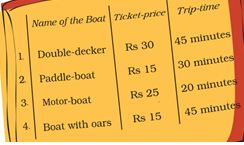1. Rs 10

2. Rs 40

3. Rs 25

4. Rs 15

7. Sita purchased a 2m glass rod for Rs.200. She gave 50cm to her friend. What amount should she take for a 50 cm long glass rod from her friend?

1. Rs 50

2. Rs 150

3. Rs 100

4. Rs 200

8. Match the following

 (a) 35 Rupees 40 Paisa (i) Rs. 105.30 (b) 365 Rupees 40 Paisa (ii) Rs. 70.99 (c) 70 Rupees 99 Paisa (iii) Rs. 365.40 (d) 105 Rupees 30 Paisa (iv) Rs. 35.40
9. Find the quotient:

1. Rs 371.25 $÷$ 5
2. Rs 252 $÷$ 4
10. A lady bought a saree and a sweater for Rs. 3489.50. If the sweater costs Rs. 743.75, what is the cost of the saree?

11. How much money Renu has if she has five Rs. 10 notes, three Rs. 50 notes and ten Rs 100 notes.

12. Praveen purchased 3 pens at the rate of Rs 14 each, 6 books at the rate of Rs 164 each, 7 maps at the rate of Rs 8 each, and 9 notebooks at the rate of Rs 17 each. Make a bill for the purchases. Also, find the total he has to pay to the shopkeeper.

13. Akhil bought 3 kg of apples at the rate of Rs 35 per kg, 3 kg of guava at the rate of Rs 16 per kg, 5 kg of grapes at the rate of Rs 40 per kg and 7 kg of oranges at the rate of Rs 25 per kg. Make a bill for the purchases. Find the total amount he has to pay to the shopkeeper.

14. From the price list find the money each person paid for the purchase of the following items:

 Items Price (in rupees) Soap 35.50 Potato chips 12.00 Biscuit 4.35 Soft drink 9.75 Candy 1.25
1. Alisha bought 1 packet of potato chips and 2 soft drinks.
2. Alka bought 3 packets of biscuits, 1 packet of chips and 4 candies.
15. Solve the following:

1. If the cost of 6 umbrellas is Rs 960 then, what is the cost of 3 umbrellas?
2. If the cost of 6 packets of biscuits is Rs 126 then, what is the cost of 10 packets of biscuits?
16. Subtract:

1. Rs. 855.75 - Rs. 323.24
2. Rs. 398.35 - Rs. 221.24
3. Rs. 3889.50 - Rs. 743.35
4. Rs. 483.18 - Rs. 238.31

CBSE Worksheet-01
Class - IV Mathematics – Money

Solution

1. Fill in the blanks with suitable words:

1. Rs. 12. Cost of 5 pen = Rs. 60
Then, Cost of one pen = $\frac{60}{5}$ = Rs. 12

2. 20
1 Rupees = 100 paise
$\frac{1}{5}$ of 1 rupees = $\frac{1×100}{5}$  = 20 paise

3. 22355.85
If the price of 1 television is 2032.35 rupees.
The Price of 11 television = 2032.35 $×$ 11  = 22355.85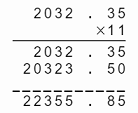4. 63 months   ( 1yr = 12 months, so 5 yr = 5 * 12 = 60 )

5. Rupee

2. State whether each of the following statement is True or False:

1. True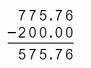2. False
Sum of Rs. 231.50, Rs. 236.22 and Rs. 333.22 is equal to Rs. 800.94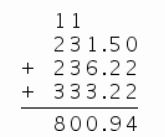3. True, In Indian currency, Rs stands for Rupees.

4. False $\frac{1}{4}$ of 400 Paisa = $\frac{1}{4}$ $×400$ = 100 Paisa = Rs. 1.

5. True
Rs 500 = 250 $×$ Rs. 2.

3. (a) Rs 497
Explanation: Amount Tom received on his birthday = Rs 517
Amount left with Tom now = Rs.20
Hence amount spent by Tom = 517 - 20 = Rs.497
4. (c) Rs 800
Explanation: Telephone bill for the month of January = Rs. 350
Telephone bill for the month of February = Rs. 450
Hence total bill for both the months =  350 + 450 = Rs. 800
5. (b) Rs 3744
Explanation:
A man saves Rs 78 per month.
1 year = 12 month
4 year = 12 × 4 = 48
Money save in 4 years= 78 × 48 = Rs 3744
6. (b) Rs 40
Explanation:
Ticket price for motor boat is Rs.25
Ticket price for oar boat is Rs. 15.
So total price paid is 25 + 15 = 40
Hence Rs.40.
7. (a) Rs 50
Explanation: Sita purchased 2m glass rod for Rs.200.
Cost of 1m glass road = 200 ÷ 2 = Rs100
1 m = 100 cm
$\frac{1}{2}$ m = 50 cm
Cost of glass road she give to her frind =  $\frac{1}{2}$ × 100 = Rs 50
8. (a) – (iv), (b) – (iii), (c) – (ii), (d) – (i)

1. Rs 371.25 $÷$ 5 = Rs 74.25
2. Rs 252 $÷$ 4 = Rs 63
9. Cost of sweater = Rs. 743.75
Total cost of saree and sweater = Rs. 3489.50
Then, the cost of saree = 3489.50 - 743.75 = 2745.75
So, the cost of saree = Rs. 2745.75

10. Five 10 rupees note = 5 $×$ 10 = 50 rupees
Three 50 rupees note = 3 $×$ 50 = 150 rupees
Ten 100 rupees note = 10 $×$ 100 = 1000 rupees
Now, the total money Renu has = 1000 + 150 + 50 = 1200 rupees

11. Cost of 3 pens = 14 $×$ 3 = Rs 42
Cost of 6 books = 6 $×$ 164 = Rs 984
Cost of 7 maps = 7 $×$ 8 = Rs 56
Cost of 9 notebooks = 9 $×$ 17 = Rs 153
now, total bill to be paid = 42 + 984 + 56 + 153 = Rs 1,235

12. Cost of apple = 35 $×$ 3 = Rs 105
Cost of Guava = 16 $×$ 3 = Rs 48
Cost of Grapes = 40 $×$ 5 = Rs 200
Cost of Oranges = 25 $×$ 7 = Rs 175
Total payment for purchase = 105 + 48 + 200 + 175 = Rs 528

1. Price of 1 potato chips packet = Rs 12
Price of 1 soft drink = Rs 9.75
then, price of 2 soft drink = 9.75 $×$ 2 = 19.50
So, bill paid by Alisha = 12 + 19.50 = Rs 31.50
2. Price of 1 packet of biscuit = Rs 4.35
then, price of 3 packets of biscuits = 4.35 $×$ 3 = Rs 13.05
Price of 1 candy = Rs 1.25
then, the price of 4 candies = 1.25 $×$ 4 = Rs 5
So, bill paid by Alka = 13.05 + 5 = Rs 18.05
1. Cost of 6 umbrella = Rs 960
now, the cost of 1 umbrella = $\frac{960}{6}$ = Rs 160
then, the cost of 3 umbrella = 160 $×$ 3 = Rs 480
2. Cost of 6 packets of biscuits = Rs 126
now, the cost of 1 packet of biscuits = $\frac{126}{6}$ = Rs 21
then, cost of 10 packets of biscuits = 21 $×$ 10 = Rs 210
1. Rs. 855.75 - Rs. 323.24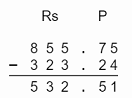2. Rs. 398.35 - Rs. 221.24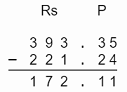3. Rs. 3889.50 - Rs. 743.35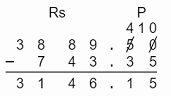4. Rs. 483.18 - Rs. 238.31